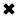# Capacitance in Series Explained

Capacitance in Series

When capacitors are connected one after each other this is called connecting in series. This is shown below.We rarely observe any mutual interaction among capacitors. We don't have to worry about mutual capacitance very often, the way we have to think about mutual inductance when working with wire coils.

When we connect two or more capacitors in series, their values combine just as resistances combine in parallel, assuming that no mutual capacitance exists among the components. If we connect two capacitors of the same value in series, the net capacitance equals half the capacitance of either component alone. In general, if we have several capacitors connected in series, we observe a net capacitance smaller than that of any of the individual components. As with resistances and inductances, we should always use the same size units when we calculate the net capacitance of any combination.

Consider several capacitors with values C1, C2, C3,..., C connected in series. We can find the net capacitance C using the formula:If we add two or more equivalent capacitances in series (connected parallel), and if one of them has a value many times totally smaller than the values of all the others, then the net capacitance equals the smallest capacitance for most practical charging purposes.

Problem 11-1

Suppose that two capacitances, C1 = 0.10 iF and C2 = 0.050 .tF, appear in series as shown in Fig. 11-3. What's the net capacitance?Fig. 11-3. Capacitors in Series.

Why the Curved Line?

Do you wonder why the capacitor symbols in Fig. 11-3 consist of one single line and one curved line? Engineers commonly use this noation because, in many situations, one end of the capacitor (the "curved-line" end) connects directly to, or faces toward, a common ground point (a neutral point, with a reference voltage of zero). In the circuit of Fig. 11-3, no common ground exists, so it doesn't matter which way the capacitors go.

##### Basic Electricity Handbook, Vol. 1• FULLY ILLUSTRATED
• GREAT FOR SCHOOL PROJECTS
• GREAT PRICE: \$5.99
• ...

This 100+ page e-book is a great guide for those who have a basic interest in the field of electricity. This well-illustrated e-book, coupled with some basic knowledge of electricity, will give you a broad theoretical background in this fundamental subject.

CONTENTS
• FUNDAMENTALS OF ELECTRICITY
• HOW DOES ELECTRICITY WORK?
• HOW IS ELECTRICITY GENERATED?
• WHERE DOES ELECTRICITY COME FROM?
• BASIC HOME WIRING
• ELECTRICITY FOR STUDENTS
• ENERGY SAVING TIPS

##### TRAINING EF COURSES

Content Community Connection
Top